How to Calculate Sample Size in Randomized Controlled Trial?
 Practical Biostatistics How to Calculate Sample Size in Randomized Controlled Trial? Baoliang Zhong, MD From A ffiliated Mental Health Center, Tongji Medical College of Huazhong University of Science & Technology Corresponding to: Dr. Baoliang Zhong, MD, Affiliated Mental Health Center, Tongji Medical College of Huazhong University of Science & Technology. No. 70, Youyi Road, Qiaokou District, Wuhan,430022,P R China. Tel/fax: 15071307899. Email: pkuhaizhilan@hotmail.com Abstract To design clinical trials, efficiency, ethics, cost effectively, research duration and sample size calculations are the key things to remember. This review highlights the statistical issues to estimate the sample size requirement. It elaborates the theory, methods and steps for the sample size calculation in randomized controlled trials. It also emphasizes that researchers should consider the study design first and then choose appropriate sample size calculation method. Key words Randomized Controlled Trial; Sample Size Calculation J Thorac Dis 2009;1:51-54. DOI: 10.3978/j.issn.2072-1439.2009.12.01.011 Introduction Randomized controlled trial (RCT) is considered as the gold standard for evaluating intervention or health care. Compared with an observational study, randomization is an effective method to balance confounding factors between treatment groups and it can eliminate the influence of confounding variables. When a research investigator wants to design a clinical trial, the key consideration is to know how many participants are to be added to the sample to obtain significant results for the study. Even the most rigorously executed study may fail to answer its research question if the sample size is too small. On the other hand, study with large samples will be more difficult to carry out and it will not be cost effective. The goal of sample size estimation is to calculate an appropriate number of subjects for a given study design (1). Four statistical conceptions of sample size calculation in RCT design (2). The null hypothesis and alternative hypothesis. In statistical hypothesis testing, the null hypothesis set out for a particular significance test and it always occurs in conjunction with an alternative hypothesis. The null hypothesis is set up to be rejected, thus if we want to compare two interventions, the null hypothesis will be “there is no difference”versus the alternative hypothesis of “there is a difference”. However, not being able to reject the null hypothesis does not mean that that it is true, it just means that we do not have enough evidence to reject the null hypothesis. α/type I error In classical statistical terms, type I error is always associated with the null hypothesis. From the probability theory prospective, there’s no such thing as “my results are right” but rather “how much error I am committing”.The probability of committing a type I error (rejecting the null hypothesis when it is actually true) is called α (alpha). For example, we predefined a statistical significance level of α =0.05, a positive P value equaled 0.03 was found at the end of a completed two-arm trial. Two possibilities for this significant difference can exist simultaneously (assuming that all bias have been controlled). One reason is that a real difference exists between the two interventions and the other reason is that this difference is by chance, but there is only 3% chance that this difference is just by chance. . Hence, if the p-value is more close to 0 then the chances of difference occurring due to “chance” are very low. To be conservative, a two-sided test is usually conducted compared to one-sided test, which requires smaller sample size. The type I error is usually set at two sided 0.05, not all, but some study design is exceptive. β/type II error As null hypothesis is associated with type I error, the alternative hypothesis is associated with type II error, when we are not able to reject the null hypothesis. This is given by the power of the research (1- type II error/β ): the probability of rejecting the null hypothesis when it is false. Conventionally, the power is set at 0.80, for higher the power, the more sample is required. Four types of comparisons in RCT design (3,4) Parallel RCT design is most commonly used, which means all participants are randomized to two (the most common) or more arms of different interventions treated concurrently. Superiority trials To verify that a new treatment is more effective than a standard treatment from a statistical point of view or from a clinical point of view, its corresponding null hypothesis is that: The new treatment is not more efficacious than the control treatment by a statistically/clinically relevant amount. Based on the nature of relevant amount, superiority design contains statistical superiority trials and clinical superiority trials. Equivalence trials The objective of this design is to ascertain that the new treatment and standard treatment are equally effective. The null hypothesis of that is: Both two treatments differ by a clinically relevant amount. Non-inferiority trials Non-inferiority trials are conducted to show that the new treatment is as effective but need not superior when compared to the standard treatment. The corresponding null hypothesis is: The new treatment is inferior to the control treatment by a clinically relevant amount. One-sided test is performed in both superiority and non-inferiority trials, and two-sided test is used in equivalence trials. The hypothesis testing of different design is summarized in Table 1. General formulas for sample size calculation (5,6). Assuming RCT has two comparison groups and both groups have the same size of subjects; sample size calculation depends on the type of primary outcome measures. Parameter definitions N=size per group; p=the response rate of standard treatment group; p0= the response rate of new drug treatment group; zx= the standard normal deviate for a one or two sided x; d= the real difference between two treatment effect;δ0= a clinically acceptable margin; S2= Polled standard deviation of both comparison groups. Dichotomous variable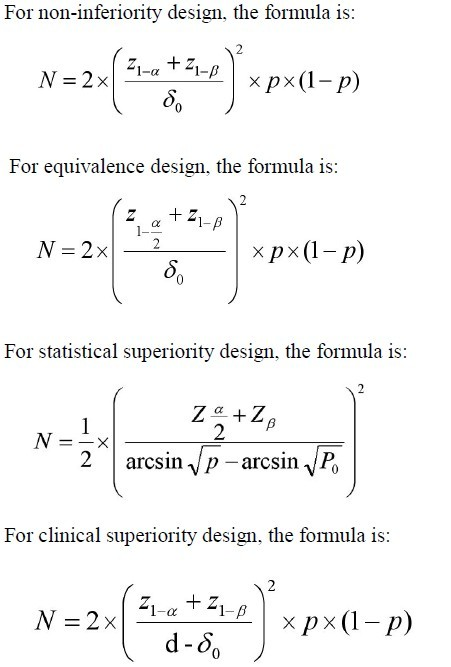Continuous variable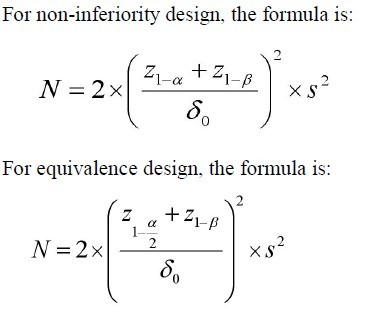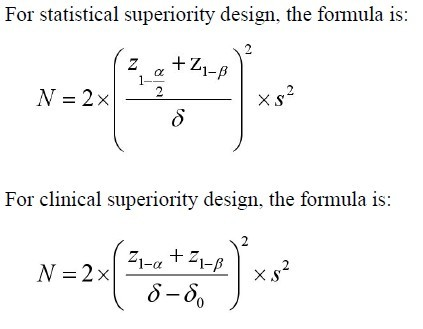Example 1: Calculating sample size when outcome measure is dichotomous variable. Problem: The research question is whether there is a difference in the efficacy of mirtazapine (new drug) and sertraline (standard drug) for the treatment of resistant depression in 6-week treatment duration. A ll parameters were assumed as follows: p =0.40; p0=0.58;α=0.05;β=0.20; δ=0.18; δ0=0.10. Parameter definitions N=size per group; p=the response rate of standard treatment group; p0= the response rate of new drug treatment group; zx= the standard normal deviate for a one or two sided x; d= the real difference between two treatment effect;δ0= a clinically acceptable margin; S2= Polled standard deviation of both comparison groups.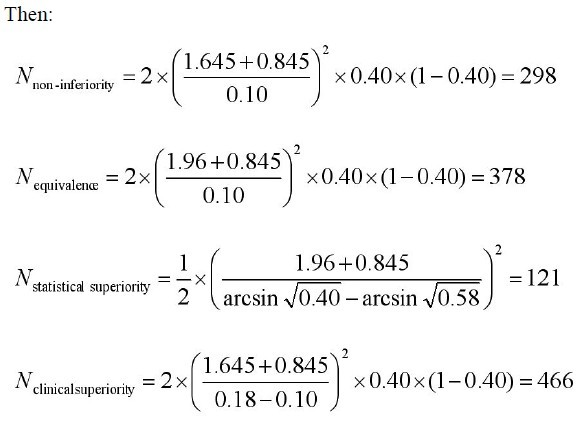Example 2: Calculating sample size when outcome measure is continuous variable. Problem: The research question is whether there is a difference in the efficacy of A CE II antagonist (new drug) and A CE inhibitor (standard drug) for the treatment of primary hypertension. Change of sitting diastolic blood pressure (SDBP, mmHg) is the primary measurement, compared to baseline. All parameters were assumed as follows: mean change of SDBP in new drug treatment group=18 mm Hg; mean change of SDBP in standard treatment group =14 mm Hg;α=0.05;β=0.20; δ=4 mmHg; δ0=3 mm Hg; s=8mm Hg.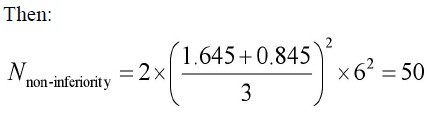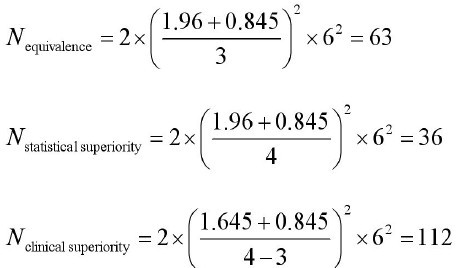Discussion Indeed, the steps for calculating sample size mirror the steps required for designing a RCT. Firstly, the researcher should specify the null and alternative hypotheses, along with the type I error rate and the power (1- type II error rate). Secondly, the researcher can gather the data of relevant parameters of interest but sometimes a pilot study may be required. Thirdly, the sample size can be estimated based on several reasonable parameters. In fact the key point which readers need to know is about the choice of null and alternative hypothesis, which should be adjusted according the study objective. Some readers might encounter obstacle in the determination of non-inferiority/equivalence/superiority margin. This parameter has clinical significance, which should be cautiously determined and it must be reasonable. Sometimes, if δ is too large, several inefficacious drugs will appear in the market for they can be judged as non-inferiority/equivalence; On the contrary, if δ is too small, some potential useful drugs will be neglected. In short, the choosing of δ is based on the explicit discussion of clinical experts and statisticians, not only depends on statisticians’suggestion. The other important thing to remember is that when δ is determined finally, it cannot be changed (7). Conclusions This paper gives simple introduction of principals and methods of sample size calculation. A researcher can calculate the sample size given the types of design and measures of outcome mentioned above. It also provides some knowledge on what information will be needed when coming to consult a biostatistician for sample size determination. If someone is interested in designing a non-inferior- ity/equivalence/superiority RCT, a consultation from a biostatistician is recommended. References Hulley SB. Designing Clinical Research: An Epidemiologic Approach (3rd ed.). Philadelphia:Wolters Kluwer Health, 2007. Wittes J.Sample size calculations for randomized controlled trials. Epidemiol Rev 2002;24:39-53.[LinkOut] Lesaffre E. Superiority, equivalence, and non-inferiority trials. Bull NYU Hosp Jt Dis 2008;66:150-4.[LinkOut] ICH Harmonised Tripartite Guideline. Statistical principles for clinical trials. Available from: http://www.ich.org/pdfICH/e9.pdf,1998.[LinkOut] Hwang IK, Morikawa T. Design issues in noninferiority/equivalence trials. Drug Information J 1999;33:1205-18. Li LM, Ye DQ, Zhan SY. Epidemiology (6th ed.) Beijing: People's medical publishing house, 2007. Liu YX, Yao C, Chen F, Chen QG, Su BH, Sun RY. Statistical Methods in Clinical Noninferiority/Equivalence Evaluation. Chin J Clin Pharmacol Ther 2000;5:344-9. Cite this article as: Zhong BL. How to Calculate Sample Size in Randomized Controlled Trial? J Thorac Dis 2009;1:51-54. doi: 10.3978/j.issn.2072-1439.2009.12.01.011